# COP 4710 Database Systems Spring 2004 Day 9

• Slides: 45COP 4710: Database Systems Spring 2004 -Day 9 – February 2, 2004 – Introduction to Functional Dependencies Instructor : Mark Llewellyn [email protected] ucf. edu CC 1 211, 823 -2790 http: //www. cs. ucf. edu/courses/cop 4710/spr 2004 School of Electrical Engineering and Computer Science University of Central Florida COP 4710: Database Systems (Day 9) Page 1 Mark Llewellyn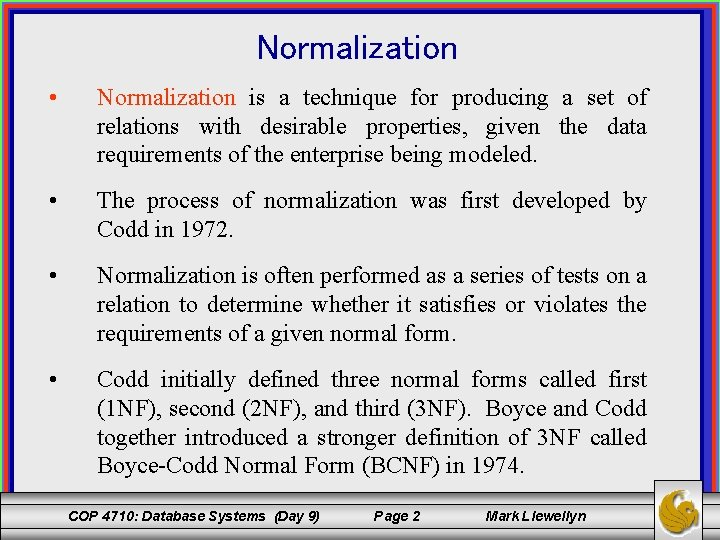Normalization • Normalization is a technique for producing a set of relations with desirable properties, given the data requirements of the enterprise being modeled. • The process of normalization was first developed by Codd in 1972. • Normalization is often performed as a series of tests on a relation to determine whether it satisfies or violates the requirements of a given normal form. • Codd initially defined three normal forms called first (1 NF), second (2 NF), and third (3 NF). Boyce and Codd together introduced a stronger definition of 3 NF called Boyce-Codd Normal Form (BCNF) in 1974. COP 4710: Database Systems (Day 9) Page 2 Mark Llewellyn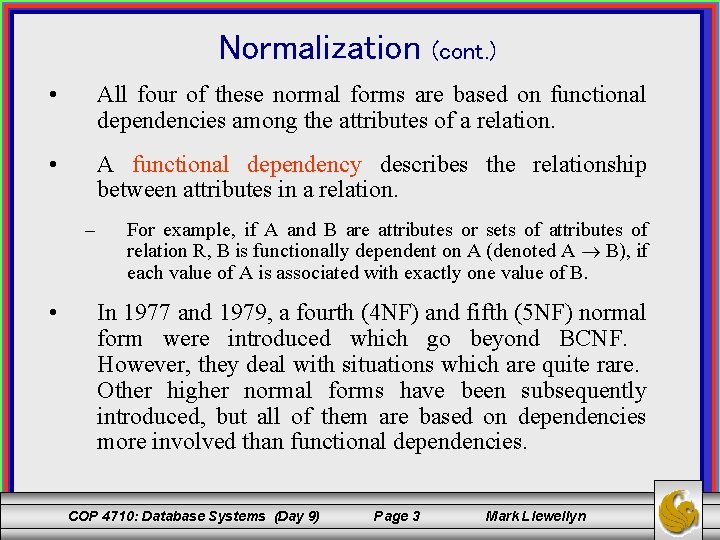Normalization (cont. ) • All four of these normal forms are based on functional dependencies among the attributes of a relation. • A functional dependency describes the relationship between attributes in a relation. – • For example, if A and B are attributes or sets of attributes of relation R, B is functionally dependent on A (denoted A B), if each value of A is associated with exactly one value of B. In 1977 and 1979, a fourth (4 NF) and fifth (5 NF) normal form were introduced which go beyond BCNF. However, they deal with situations which are quite rare. Other higher normal forms have been subsequently introduced, but all of them are based on dependencies more involved than functional dependencies. COP 4710: Database Systems (Day 9) Page 3 Mark Llewellyn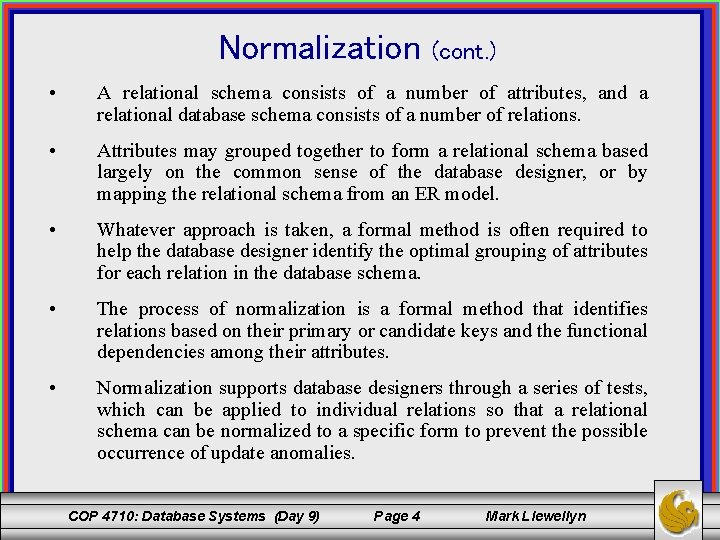Normalization (cont. ) • A relational schema consists of a number of attributes, and a relational database schema consists of a number of relations. • Attributes may grouped together to form a relational schema based largely on the common sense of the database designer, or by mapping the relational schema from an ER model. • Whatever approach is taken, a formal method is often required to help the database designer identify the optimal grouping of attributes for each relation in the database schema. • The process of normalization is a formal method that identifies relations based on their primary or candidate keys and the functional dependencies among their attributes. • Normalization supports database designers through a series of tests, which can be applied to individual relations so that a relational schema can be normalized to a specific form to prevent the possible occurrence of update anomalies. COP 4710: Database Systems (Day 9) Page 4 Mark Llewellyn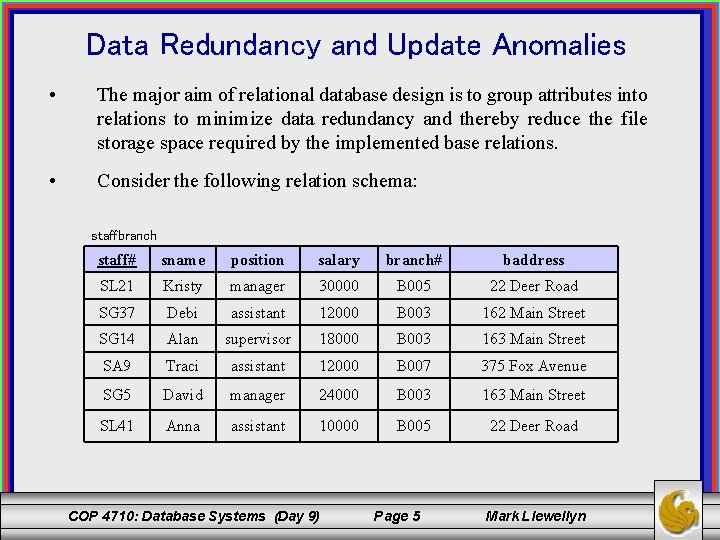Data Redundancy and Update Anomalies • The major aim of relational database design is to group attributes into relations to minimize data redundancy and thereby reduce the file storage space required by the implemented base relations. • Consider the following relation schema: staffbranch staff# sname position salary branch# baddress SL 21 Kristy manager 30000 B 005 22 Deer Road SG 37 Debi assistant 12000 B 003 162 Main Street SG 14 Alan supervisor 18000 B 003 163 Main Street SA 9 Traci assistant 12000 B 007 375 Fox Avenue SG 5 David manager 24000 B 003 163 Main Street SL 41 Anna assistant 10000 B 005 22 Deer Road COP 4710: Database Systems (Day 9) Page 5 Mark Llewellyn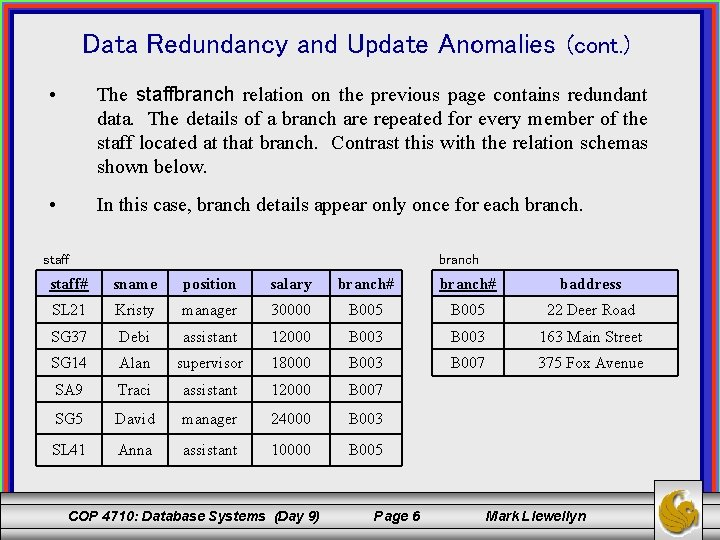Data Redundancy and Update Anomalies (cont. ) • The staffbranch relation on the previous page contains redundant data. The details of a branch are repeated for every member of the staff located at that branch. Contrast this with the relation schemas shown below. • In this case, branch details appear only once for each branch. staff branch staff# sname position salary branch# baddress SL 21 Kristy manager 30000 B 005 22 Deer Road SG 37 Debi assistant 12000 B 003 163 Main Street SG 14 Alan supervisor 18000 B 003 B 007 375 Fox Avenue SA 9 Traci assistant 12000 B 007 SG 5 David manager 24000 B 003 SL 41 Anna assistant 10000 B 005 COP 4710: Database Systems (Day 9) Page 6 Mark LlewellynData Redundancy and Update Anomalies (cont. ) • Relations which contain redundant data may have problems called update anomalies, which can be classified as insertion, deletion, or modification (update) anomalies. Update Anomalies 1. To insert the details of new staff members into the staffbranch relation, we must include the details of the branch at which the new staff member is to be located. • 2. For example, if the new staff member is to be located at branch B 007, we must enter the correct address so that it matches existing tuples in the relation. The database schema with staff and branch does not suffer this problem. To insert the details of a new branch that currently has no staff members, we’ll need to insert null values for the attributes of the staff such as staff number. However, since staff number is a primary key, this would violate key integrity and is not allowed. Thus we cannot enter information for a new branch with no staff members! COP 4710: Database Systems (Day 9) Page 7 Mark Llewellyn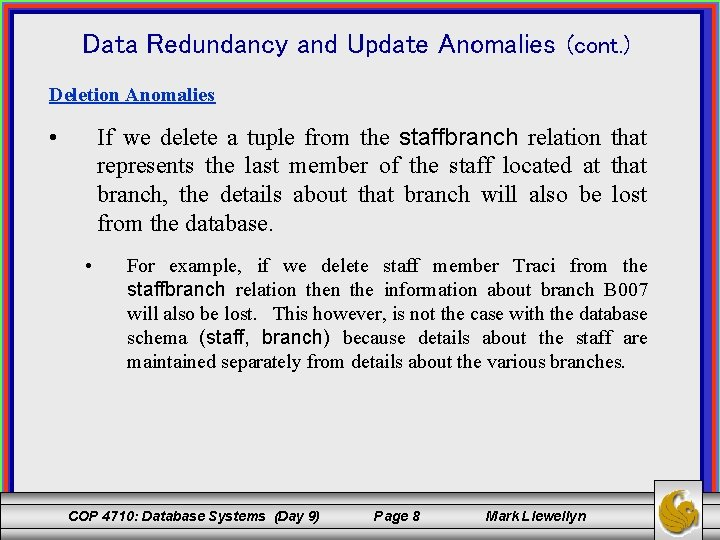Data Redundancy and Update Anomalies (cont. ) Deletion Anomalies • If we delete a tuple from the staffbranch relation that represents the last member of the staff located at that branch, the details about that branch will also be lost from the database. • For example, if we delete staff member Traci from the staffbranch relation the information about branch B 007 will also be lost. This however, is not the case with the database schema (staff, branch) because details about the staff are maintained separately from details about the various branches. COP 4710: Database Systems (Day 9) Page 8 Mark Llewellyn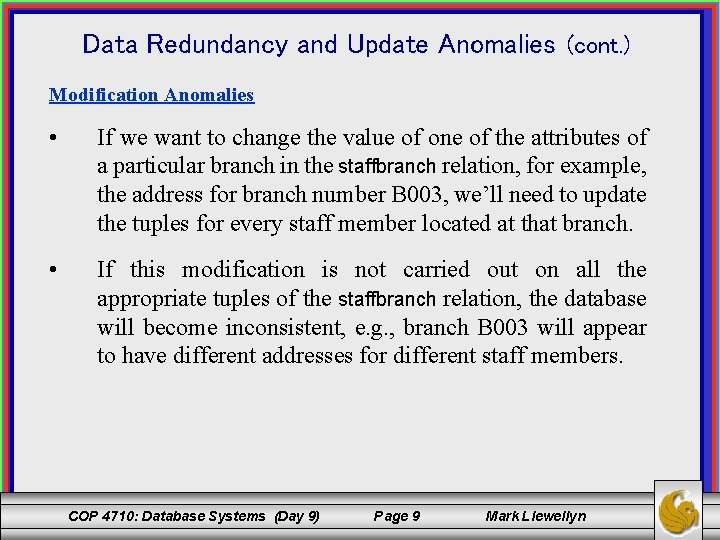Data Redundancy and Update Anomalies (cont. ) Modification Anomalies • If we want to change the value of one of the attributes of a particular branch in the staffbranch relation, for example, the address for branch number B 003, we’ll need to update the tuples for every staff member located at that branch. • If this modification is not carried out on all the appropriate tuples of the staffbranch relation, the database will become inconsistent, e. g. , branch B 003 will appear to have different addresses for different staff members. COP 4710: Database Systems (Day 9) Page 9 Mark LlewellynData Redundancy and Update Anomalies (cont. ) • The examples of three types of update anomalies suffered by the staffbranch relation demonstrate that its decomposition into the staff and branch relations avoids such anomalies. • There are two important properties associated with the decomposition of a larger relation into a set of smaller relations. 1. The lossless-join property ensures that any instance of the original relation can be identified from corresponding instances of the smaller relations. 2. The dependency preservation property ensures that a constraint on the original relation can be maintained by simply enforcing some constraint on each of the smaller relations. In other words, the smaller relations do not need to be joined together to check if a constraint on the original relation is violated. COP 4710: Database Systems (Day 9) Page 10 Mark LlewellynThe Lossless Join Property • Consider the following relation schema SP and its decomposition into two schemas S 1 and S 2. SP S 1 S 2 s# p# qty s# qty p# qty S 1 P 1 10 S 1 10 P 1 10 S 2 P 2 50 S 2 50 P 2 50 S 3 P 3 10 S 3 10 P 3 10 s# p# qty S 1 P 1 10 S 1 P 3 10 S 2 P 2 10 S 3 P 1 10 S 3 P 3 10 COP 4710: Database Systems (Day 9) These are extraneous tuples which did not appear in the original relation. However, now we can’t tell which are valid and which aren’t. Once the decomposition occurs the original SP relation is lost. Page 11 Mark Llewellyn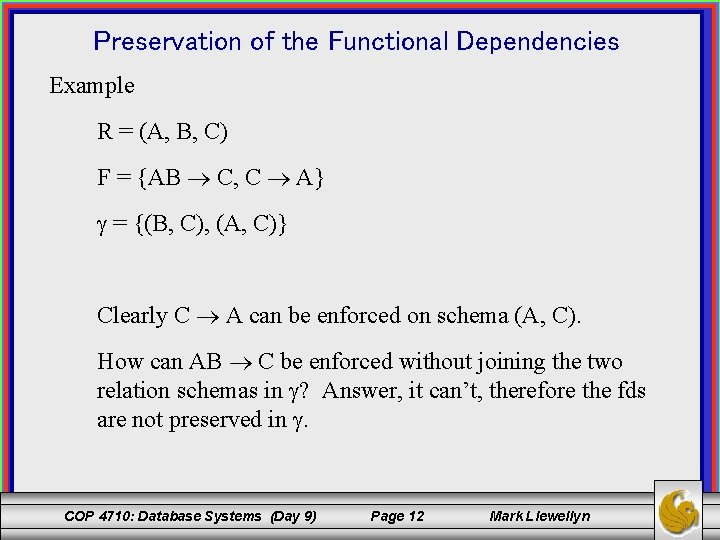Preservation of the Functional Dependencies Example R = (A, B, C) F = {AB C, C A} = {(B, C), (A, C)} Clearly C A can be enforced on schema (A, C). How can AB C be enforced without joining the two relation schemas in ? Answer, it can’t, therefore the fds are not preserved in . COP 4710: Database Systems (Day 9) Page 12 Mark Llewellyn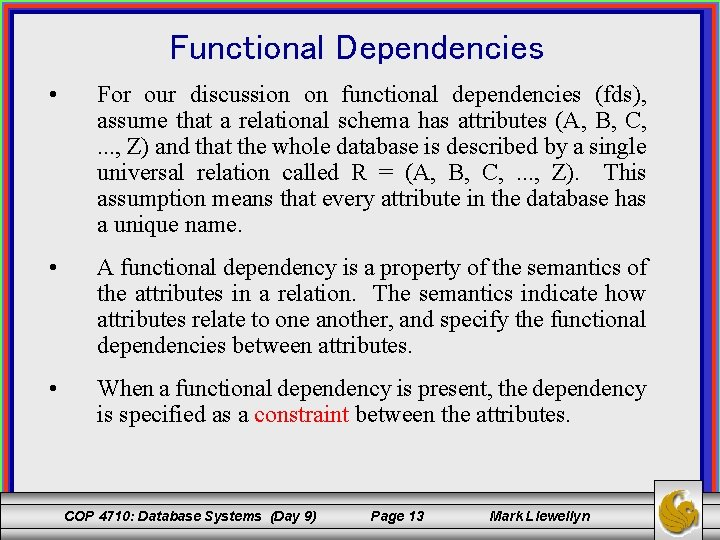Functional Dependencies • For our discussion on functional dependencies (fds), assume that a relational schema has attributes (A, B, C, . . . , Z) and that the whole database is described by a single universal relation called R = (A, B, C, . . . , Z). This assumption means that every attribute in the database has a unique name. • A functional dependency is a property of the semantics of the attributes in a relation. The semantics indicate how attributes relate to one another, and specify the functional dependencies between attributes. • When a functional dependency is present, the dependency is specified as a constraint between the attributes. COP 4710: Database Systems (Day 9) Page 13 Mark Llewellyn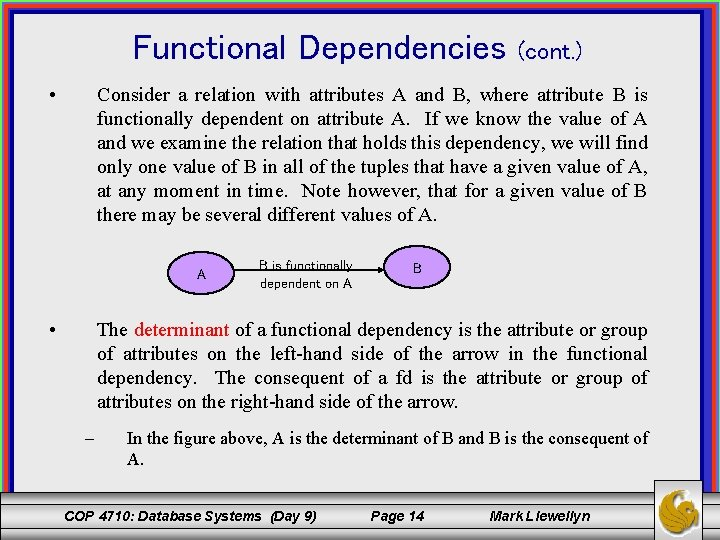Functional Dependencies • (cont. ) Consider a relation with attributes A and B, where attribute B is functionally dependent on attribute A. If we know the value of A and we examine the relation that holds this dependency, we will find only one value of B in all of the tuples that have a given value of A, at any moment in time. Note however, that for a given value of B there may be several different values of A. A • B is functionally dependent on A B The determinant of a functional dependency is the attribute or group of attributes on the left-hand side of the arrow in the functional dependency. The consequent of a fd is the attribute or group of attributes on the right-hand side of the arrow. – In the figure above, A is the determinant of B and B is the consequent of A. COP 4710: Database Systems (Day 9) Page 14 Mark LlewellynIdentifying Functional Dependencies • Look back at the staff relation on page 6. The functional dependency staff# position clearly holds on this relation instance. However, the reverse functional dependency position staff# clearly does not hold. – The relationship between staff# and position is 1: 1 – for each staff member there is only one position. On the other hand, the relationship between position and staff# is 1: M – there are several staff numbers associated with a given position. staff# position is functionally position dependent on staff# position • staff# is NOT functionally dependent on position staff# For the purposes of normalization we are interested in identifying functional dependencies between attributes of a relation that have a 1: 1 relationship. COP 4710: Database Systems (Day 9) Page 15 Mark Llewellyn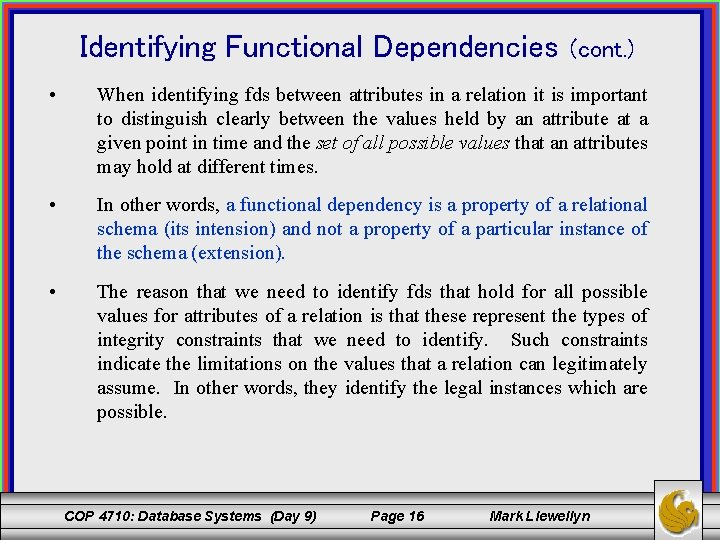Identifying Functional Dependencies (cont. ) • When identifying fds between attributes in a relation it is important to distinguish clearly between the values held by an attribute at a given point in time and the set of all possible values that an attributes may hold at different times. • In other words, a functional dependency is a property of a relational schema (its intension) and not a property of a particular instance of the schema (extension). • The reason that we need to identify fds that hold for all possible values for attributes of a relation is that these represent the types of integrity constraints that we need to identify. Such constraints indicate the limitations on the values that a relation can legitimately assume. In other words, they identify the legal instances which are possible. COP 4710: Database Systems (Day 9) Page 16 Mark LlewellynIdentifying Functional Dependencies (cont. ) • Let’s identify the functional dependencies that hold using the relation schema staffbranch shown on page 5 as an example. • In order to identify the time invariant fds, we need to clearly understand the semantics of the various attributes in each of the relation schemas in question. – For example, if we know that a staff member’s position and the branch at which they are located determines their salary. There is no way of knowing this constraint unless you are familiar with the enterprise, but this is what the requirements analysis phase and the conceptual design phase are all about! staff# sname, position, salary, branch#, baddress branch# baddress branch#, position salary baddress, position salary COP 4710: Database Systems (Day 9) Page 17 Mark Llewellyn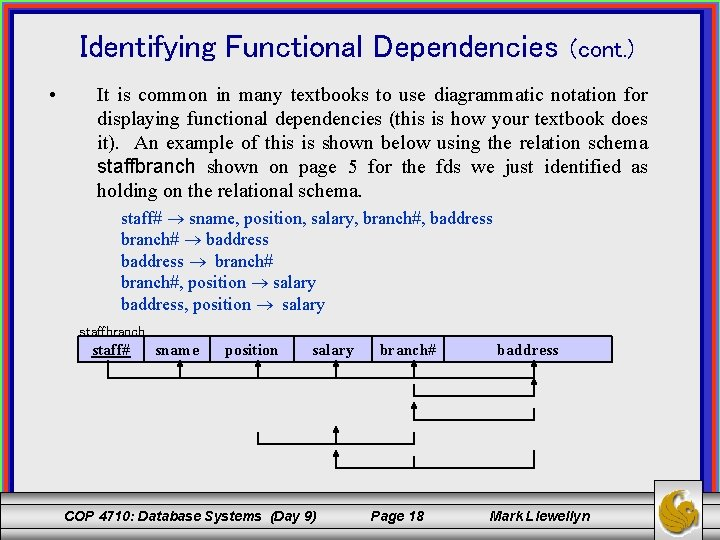Identifying Functional Dependencies • (cont. ) It is common in many textbooks to use diagrammatic notation for displaying functional dependencies (this is how your textbook does it). An example of this is shown below using the relation schema staffbranch shown on page 5 for the fds we just identified as holding on the relational schema. staff# sname, position, salary, branch#, baddress branch# baddress branch#, position salary baddress, position salary staffbranch staff# sname position salary COP 4710: Database Systems (Day 9) branch# Page 18 baddress Mark Llewellyn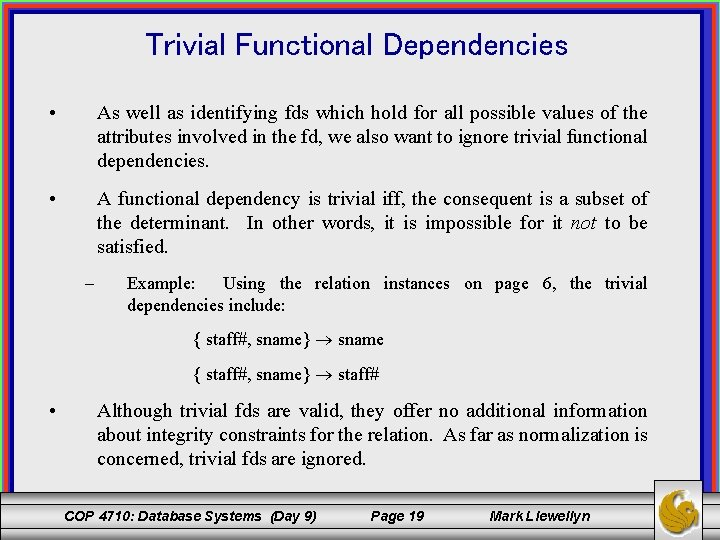Trivial Functional Dependencies • As well as identifying fds which hold for all possible values of the attributes involved in the fd, we also want to ignore trivial functional dependencies. • A functional dependency is trivial iff, the consequent is a subset of the determinant. In other words, it is impossible for it not to be satisfied. – Example: Using the relation instances on page 6, the trivial dependencies include: { staff#, sname} sname { staff#, sname} staff# • Although trivial fds are valid, they offer no additional information about integrity constraints for the relation. As far as normalization is concerned, trivial fds are ignored. COP 4710: Database Systems (Day 9) Page 19 Mark Llewellyn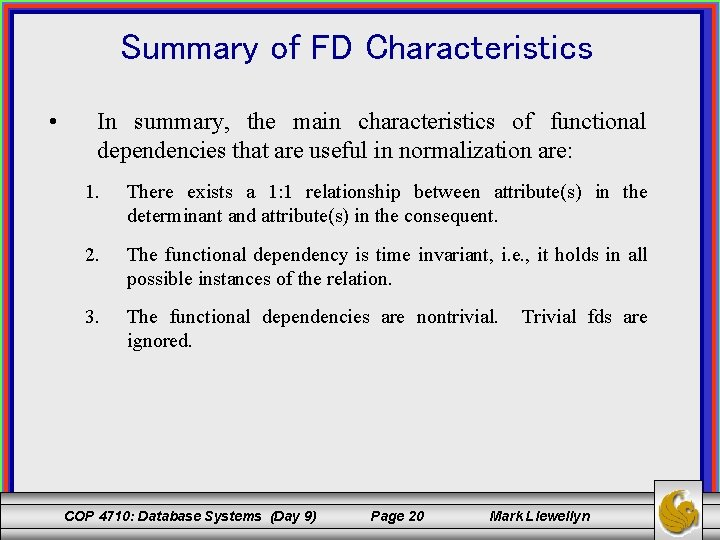Summary of FD Characteristics • In summary, the main characteristics of functional dependencies that are useful in normalization are: 1. There exists a 1: 1 relationship between attribute(s) in the determinant and attribute(s) in the consequent. 2. The functional dependency is time invariant, i. e. , it holds in all possible instances of the relation. 3. The functional dependencies are nontrivial. ignored. COP 4710: Database Systems (Day 9) Page 20 Trivial fds are Mark Llewellyn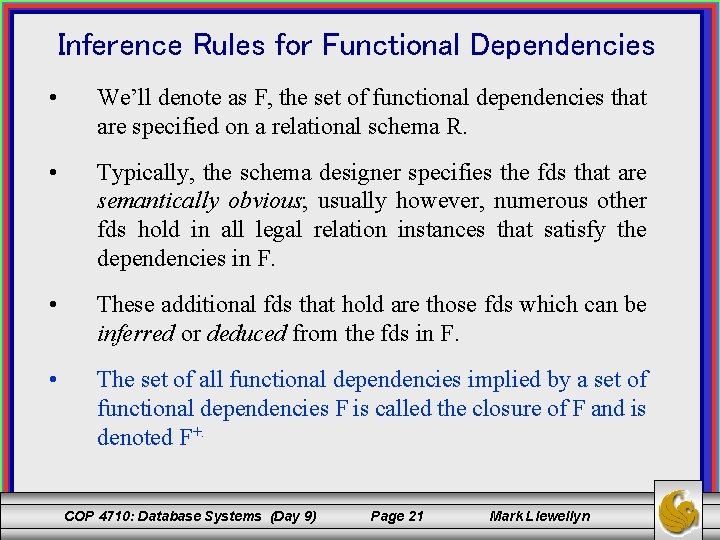Inference Rules for Functional Dependencies • We’ll denote as F, the set of functional dependencies that are specified on a relational schema R. • Typically, the schema designer specifies the fds that are semantically obvious; usually however, numerous other fds hold in all legal relation instances that satisfy the dependencies in F. • These additional fds that hold are those fds which can be inferred or deduced from the fds in F. • The set of all functional dependencies implied by a set of functional dependencies F is called the closure of F and is denoted F+. COP 4710: Database Systems (Day 9) Page 21 Mark LlewellynInference Rules (cont. ) • The notation: F ⊨ X Y denotes that the functional dependency X Y is implied by the set of fds F. • Formally, F+ {X Y | F ⊨ X Y } • A set of inference rules is required to infer the set of fds in F+. – • For example, if I tell you that Kristi is older than Debi and that Debi is older than Traci, you are able to infer that Kristi is older than Traci. How did you make this inference? Without thinking about it or maybe knowing about it, you utilized a transitivity rule to allow you to make this inference. The next page illustrates a set of six well-known inference rules that apply to functional dependencies. COP 4710: Database Systems (Day 9) Page 22 Mark Llewellyn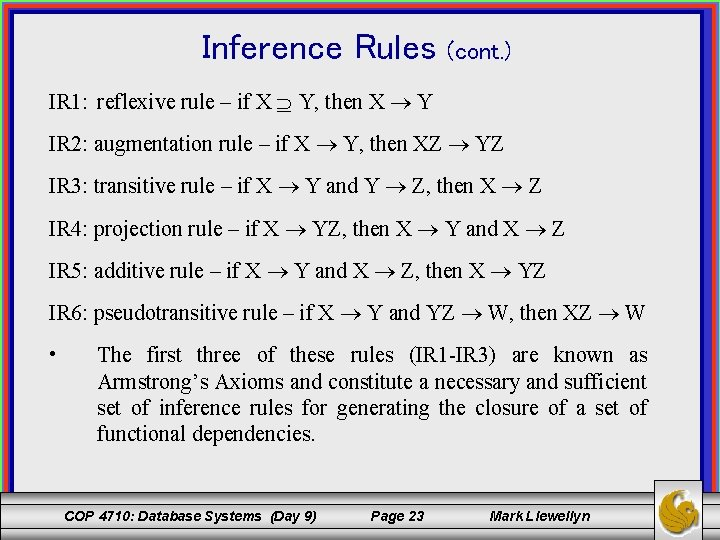Inference Rules (cont. ) IR 1: reflexive rule – if X Y, then X Y IR 2: augmentation rule – if X Y, then XZ YZ IR 3: transitive rule – if X Y and Y Z, then X Z IR 4: projection rule – if X YZ, then X Y and X Z IR 5: additive rule – if X Y and X Z, then X YZ IR 6: pseudotransitive rule – if X Y and YZ W, then XZ W • The first three of these rules (IR 1 -IR 3) are known as Armstrong’s Axioms and constitute a necessary and sufficient set of inference rules for generating the closure of a set of functional dependencies. COP 4710: Database Systems (Day 9) Page 23 Mark LlewellynExample Proof Using Inference Rules • Given R = (A, B, C, D, E, F, G, H, I, J) and F = {AB E, AG J, BE I, E G, GI H} does F ⊨ AB GH? Proof 1. 2. 3. 4. 5. 6. 7. 8. 9. 10. 11. 12. Practice Problem AB E, given in F Using the same set F, prove AB AB, reflexive rule IR 1 that F ⊨ BE H AB B, projective rule IR 4 from step 2 AB BE, additive rule IR 5 from steps 1 and 3 Answer: in next set of notes BE I, given in F AB I, transitive rule IR 3 from steps 4 and 5 E G, given in F AB G, transitive rule IR 3 from steps 1 and 7 AB GI, additive rule IR 5 from steps 6 and 8 GI H, given in F AB H, transitive rule IR 3 from steps 9 and 10 AB GH, additive rule IR 5 from steps 8 and 11 - proven COP 4710: Database Systems (Day 9) Page 24 Mark Llewellyn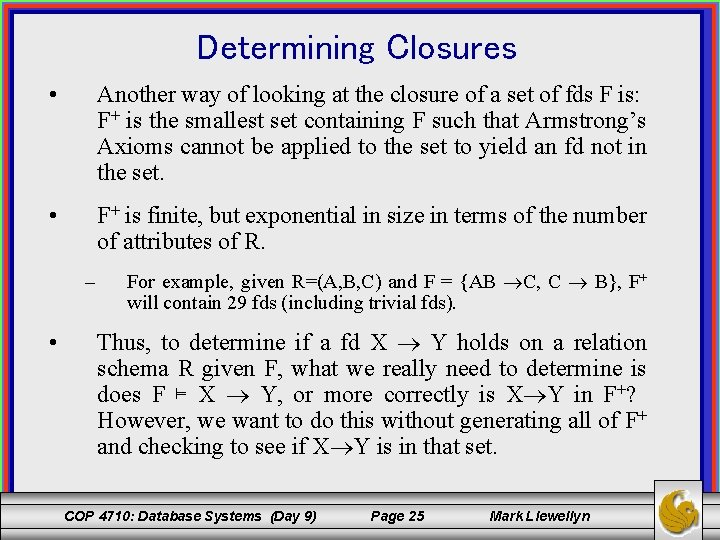Determining Closures • Another way of looking at the closure of a set of fds F is: F+ is the smallest set containing F such that Armstrong’s Axioms cannot be applied to the set to yield an fd not in the set. • F+ is finite, but exponential in size in terms of the number of attributes of R. – • For example, given R=(A, B, C) and F = {AB C, C B}, F+ will contain 29 fds (including trivial fds). Thus, to determine if a fd X Y holds on a relation schema R given F, what we really need to determine is does F ⊨ X Y, or more correctly is X Y in F+? However, we want to do this without generating all of F+ and checking to see if X Y is in that set. COP 4710: Database Systems (Day 9) Page 25 Mark Llewellyn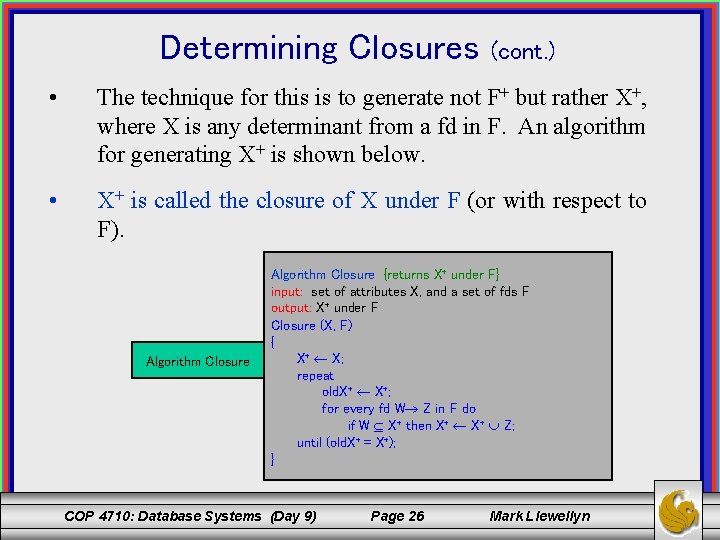Determining Closures (cont. ) • The technique for this is to generate not F+ but rather X+, where X is any determinant from a fd in F. An algorithm for generating X+ is shown below. • X+ is called the closure of X under F (or with respect to F). Algorithm Closure {returns X+ under F} input: set of attributes X, and a set of fds F output: X+ under F Closure (X, F) { X+ X; repeat old. X+ X+; for every fd W Z in F do if W X+ then X+ Z; until (old. X+ = X+); } COP 4710: Database Systems (Day 9) Page 26 Mark LlewellynExample Using Algorithm Closure Given F = {A D, AB E, BI E, CD I, E C}, Find (AE)+ pass 1 X+ = {A, E} using A D, A X+, so add D to X+, X+ = {A, E, D} using AB E, no using BI E, no using CD I, no using E C, E X+, so add C to X+, X+ = {A, E, D, C} changes occurred to X+ so another pass is required pass 2 X+ = {A, E, D, C} using A D, yes, but no changes using AB E, no using BI E, no using CD I, CD X+, so add I to X+, X+ = {A, E, D, C, I} using E C, yes, but no changes occurred to X+ so another pass is required COP 4710: Database Systems (Day 9) Page 27 Mark LlewellynExample Using Algorithm Closure Continues pass 3 X+ = {A, E, D, C, I} using A D, yes, but no changes using AB E, no using BI E, no using CD I, yes, but no changes using E C, yes, but no changes occurred to X+ so algorithm terminates (AE)+ = {A, E, C, D, I} This means that the following fds are in F+: AE AECDI COP 4710: Database Systems (Day 9) Page 28 Mark Llewellyn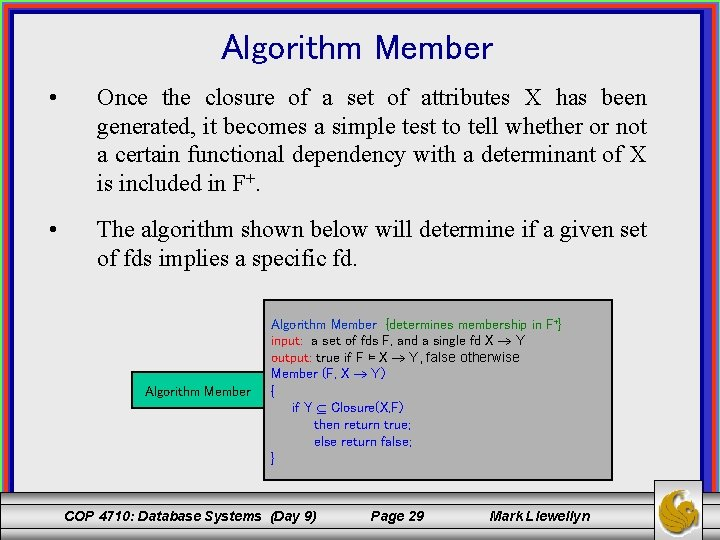Algorithm Member • Once the closure of a set of attributes X has been generated, it becomes a simple test to tell whether or not a certain functional dependency with a determinant of X is included in F+. • The algorithm shown below will determine if a given set of fds implies a specific fd. Algorithm Member {determines membership in F+} input: a set of fds F, and a single fd X Y output: true if F ⊨ X Y, false otherwise Member (F, X Y) { if Y Closure(X, F) then return true; else return false; } COP 4710: Database Systems (Day 9) Page 29 Mark Llewellyn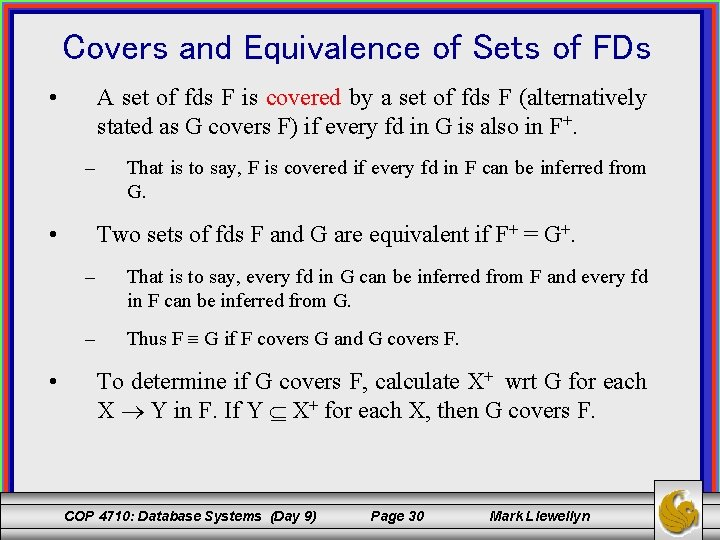Covers and Equivalence of Sets of FDs • A set of fds F is covered by a set of fds F (alternatively stated as G covers F) if every fd in G is also in F+. – • • That is to say, F is covered if every fd in F can be inferred from G. Two sets of fds F and G are equivalent if F+ = G+. – That is to say, every fd in G can be inferred from F and every fd in F can be inferred from G. – Thus F G if F covers G and G covers F. To determine if G covers F, calculate X+ wrt G for each X Y in F. If Y X+ for each X, then G covers F. COP 4710: Database Systems (Day 9) Page 30 Mark Llewellyn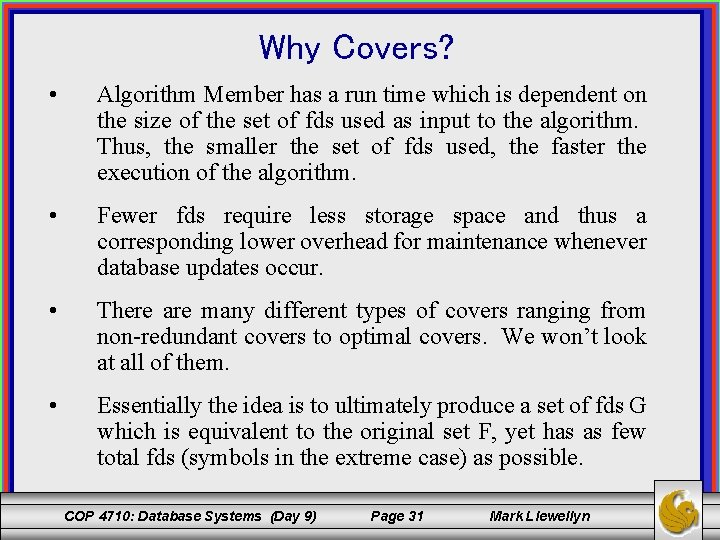Why Covers? • Algorithm Member has a run time which is dependent on the size of the set of fds used as input to the algorithm. Thus, the smaller the set of fds used, the faster the execution of the algorithm. • Fewer fds require less storage space and thus a corresponding lower overhead for maintenance whenever database updates occur. • There are many different types of covers ranging from non-redundant covers to optimal covers. We won’t look at all of them. • Essentially the idea is to ultimately produce a set of fds G which is equivalent to the original set F, yet has as few total fds (symbols in the extreme case) as possible. COP 4710: Database Systems (Day 9) Page 31 Mark LlewellynNon-redundant Covers • A set of fds is non-redundant if there is no proper subset G of F with G F. If such a G exists, F is redundant. • F is a non-redundant cover for G if F is a cover for G and F is non-redundant. Algorithm Nonredundant {produces a non-redundant cover} input: a set of fds G output: a nonredundant cover for G Nonredundant (G) { F G; for each fd X Y G do if Member(F – {X Y}, X Y) then F F – {X Y}; return (F); } COP 4710: Database Systems (Day 9) Page 32 Mark LlewellynExample: Producing a Non-redundant Cover Let G = {A B, B A, B C, A C}, find a non-redundant cover for G. F G Member({B A, B C, A C}, A B) Closure(A, {B A, B C, A C}) A+ = {A, C}, therefore A B is not redundant Member({A B, B C, A C}, B A) Closure(B, {A B, B C, A C}) B+ = {B, C}, therefore B A is not redundant Member({A B, B A, A C}, B C) Closure(B, {A B, B A, A C}) B+ = {B, A, C}, therefore B C is redundant F = F – {B C} Member({A B, B A}, A C) Closure(A, {A B, B A}) A+ = {A, B}, therefore A C is not redundant Return F = {A B, B A, A C} COP 4710: Database Systems (Day 9) Page 33 Mark Llewellyn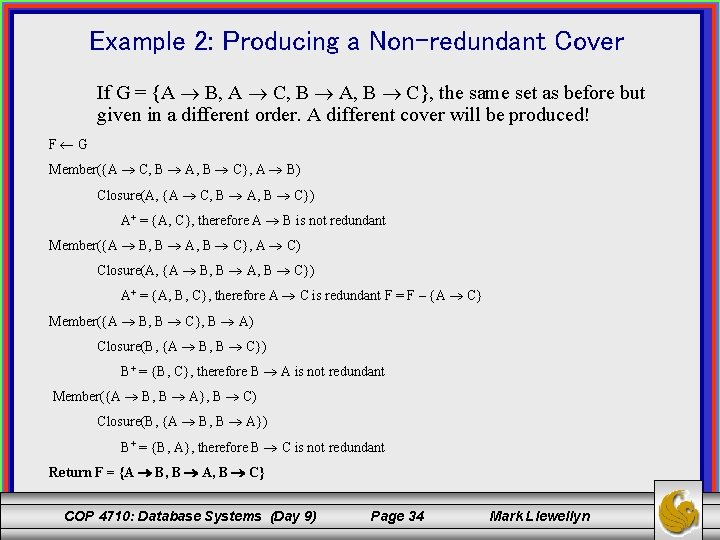Example 2: Producing a Non-redundant Cover If G = {A B, A C, B A, B C}, the same set as before but given in a different order. A different cover will be produced! F G Member({A C, B A, B C}, A B) Closure(A, {A C, B A, B C}) A+ = {A, C}, therefore A B is not redundant Member({A B, B A, B C}, A C) Closure(A, {A B, B A, B C}) A+ = {A, B, C}, therefore A C is redundant F = F – {A C} Member({A B, B C}, B A) Closure(B, {A B, B C}) B+ = {B, C}, therefore B A is not redundant Member({A B, B A}, B C) Closure(B, {A B, B A}) B+ = {B, A}, therefore B C is not redundant Return F = {A B, B A, B C} COP 4710: Database Systems (Day 9) Page 34 Mark Llewellyn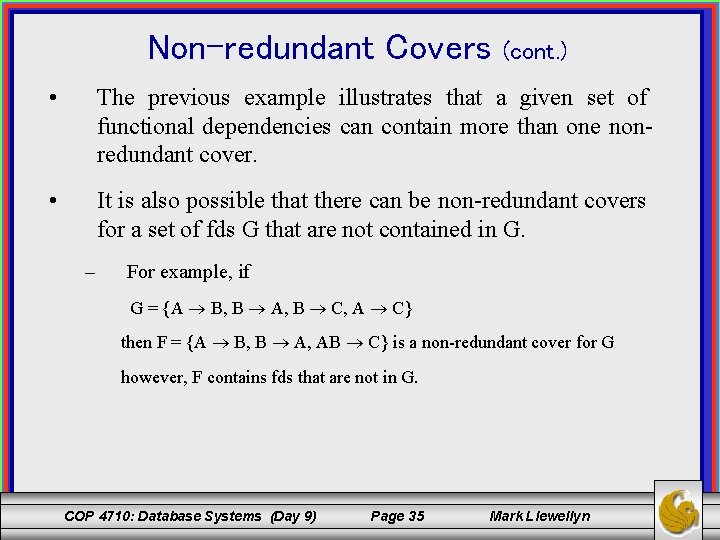Non-redundant Covers (cont. ) • The previous example illustrates that a given set of functional dependencies can contain more than one nonredundant cover. • It is also possible that there can be non-redundant covers for a set of fds G that are not contained in G. – For example, if G = {A B, B A, B C, A C} then F = {A B, B A, AB C} is a non-redundant cover for G however, F contains fds that are not in G. COP 4710: Database Systems (Day 9) Page 35 Mark Llewellyn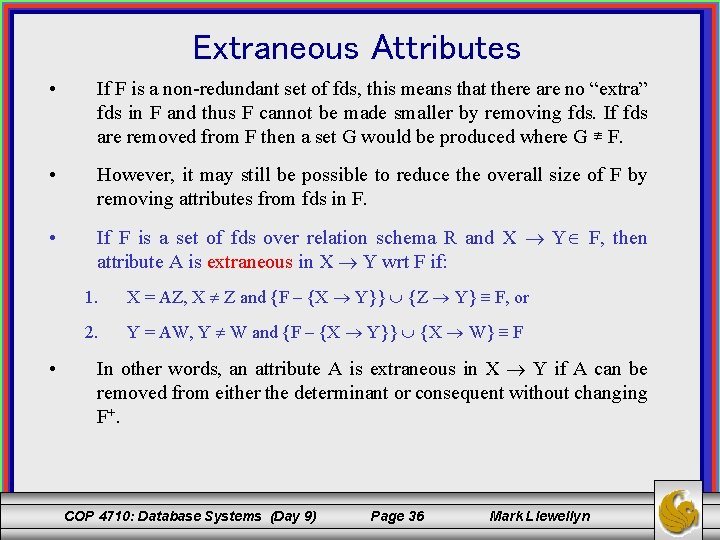Extraneous Attributes • If F is a non-redundant set of fds, this means that there are no “extra” fds in F and thus F cannot be made smaller by removing fds. If fds are removed from F then a set G would be produced where G ≢ F. • However, it may still be possible to reduce the overall size of F by removing attributes from fds in F. • If F is a set of fds over relation schema R and X Y F, then attribute A is extraneous in X Y wrt F if: • 1. X = AZ, X Z and {F – {X Y}} {Z Y} F, or 2. Y = AW, Y W and {F – {X Y}} {X W} F In other words, an attribute A is extraneous in X Y if A can be removed from either the determinant or consequent without changing F+. COP 4710: Database Systems (Day 9) Page 36 Mark Llewellyn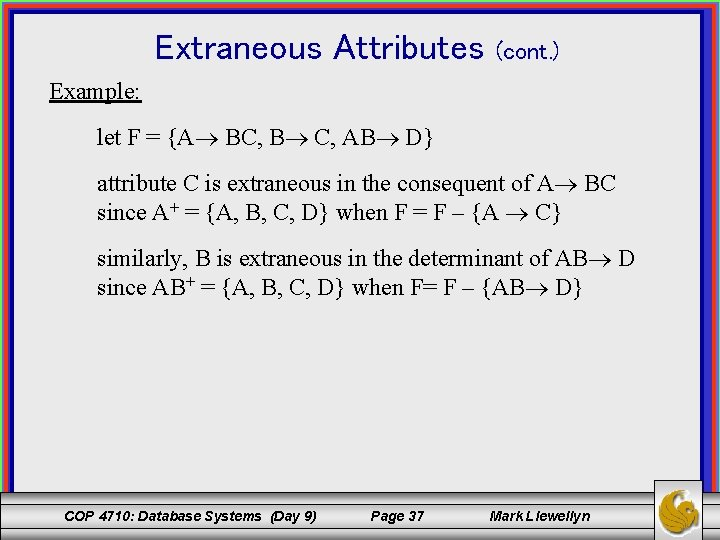Extraneous Attributes (cont. ) Example: let F = {A BC, B C, AB D} attribute C is extraneous in the consequent of A BC since A+ = {A, B, C, D} when F = F – {A C} similarly, B is extraneous in the determinant of AB D since AB+ = {A, B, C, D} when F= F – {AB D} COP 4710: Database Systems (Day 9) Page 37 Mark LlewellynLeft and Right Reduced Sets of FDs • Let F be a set of fds over schema R and let X Y F. X Y is left-reduced if X contains no extraneous attribute A. • A left-reduced functional dependency is also called a full functional dependency. X Y is right-reduced if Y contains no extraneous attribute A. X Y is reduced if it is left-reduced, right-reduced, and Y is not empty. COP 4710: Database Systems (Day 9) Page 38 Mark LlewellynAlgorithm Left-Reduce • The algorithm below produces a left-reduced set of functional dependencies. Algorithm Left-Reduce {returns left-reduced version of F} input: set of fds G output: a left-reduced cover for G Left-Reduce (G) { F G; for each fd X Y in G do for each attribute A in X do if Member(F, (X-A) Y) then remove A from X in X Y in F return(F); } COP 4710: Database Systems (Day 9) Page 39 Mark Llewellyn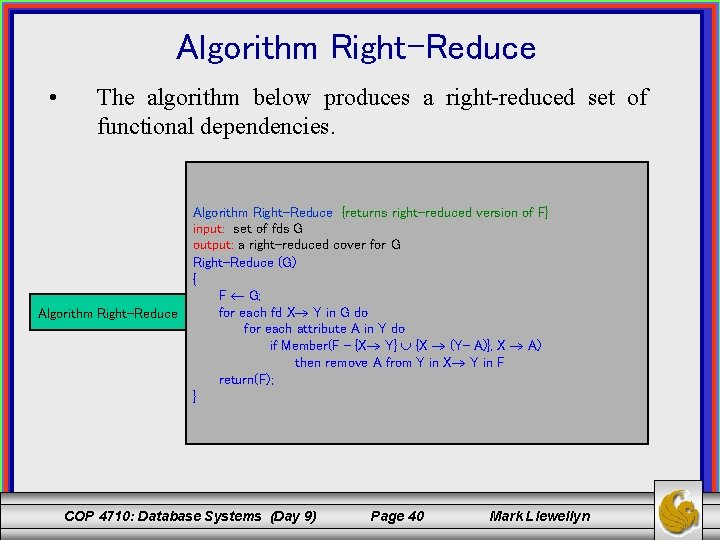Algorithm Right-Reduce • The algorithm below produces a right-reduced set of functional dependencies. Algorithm Right-Reduce {returns right-reduced version of F} input: set of fds G output: a right-reduced cover for G Right-Reduce (G) { F G; for each fd X Y in G do for each attribute A in Y do if Member(F – {X Y} {X (Y- A)}, X A) then remove A from Y in X Y in F return(F); } COP 4710: Database Systems (Day 9) Page 40 Mark LlewellynAlgorithm Reduce • The algorithm below produces a reduced set of functional dependencies. Algorithm Reduce {returns reduced version of F} input: set of fds G output: a reduced cover for G Reduce (G) { F Right-Reduce( Left-Reduce(G)); remove all fds of the form X null from F return(F); } COP 4710: Database Systems (Day 9) Page 41 If G contained a redundant fd, X Y, every attribute in Y would be extraneous and thus reduce to X null, so these need to be removed. Mark LlewellynAlgorithm Reduce • (cont. ) The order in which the reduction is done by algorithm Reduce is important. The set of fds must be left-reduced first and then right-reduced. The example below illustrates what may happen if this order is violated. Example: Let G = {B A , D A , BA D} G is right-reduced but not left-reduced. If we left-reduce G to produce F = {B A , D A , B D} We have F is left-reduced but not right-reduced! B A is extraneous on right side since B D A COP 4710: Database Systems (Day 9) Page 42 Mark Llewellyn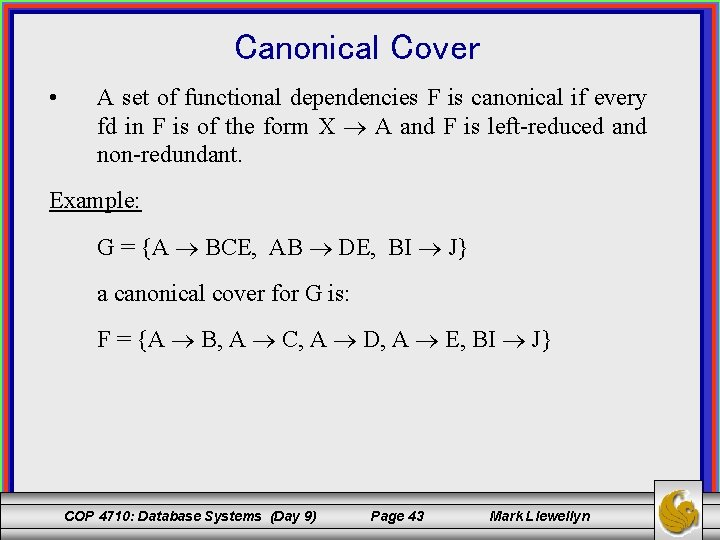Canonical Cover • A set of functional dependencies F is canonical if every fd in F is of the form X A and F is left-reduced and non-redundant. Example: G = {A BCE, AB DE, BI J} a canonical cover for G is: F = {A B, A C, A D, A E, BI J} COP 4710: Database Systems (Day 9) Page 43 Mark Llewellyn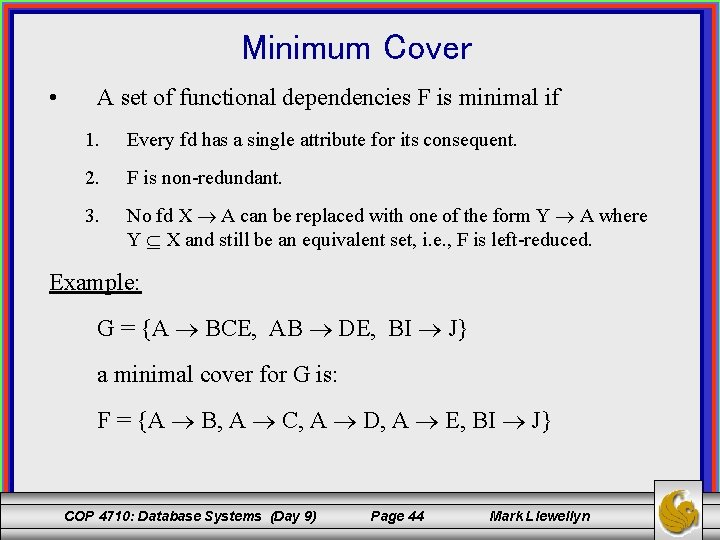Minimum Cover • A set of functional dependencies F is minimal if 1. Every fd has a single attribute for its consequent. 2. F is non-redundant. 3. No fd X A can be replaced with one of the form Y A where Y X and still be an equivalent set, i. e. , F is left-reduced. Example: G = {A BCE, AB DE, BI J} a minimal cover for G is: F = {A B, A C, A D, A E, BI J} COP 4710: Database Systems (Day 9) Page 44 Mark LlewellynAlgorithm Min. Cover • The algorithm below produces a minimal cover for a set of functional dependencies. Algorithm Min. Cover {returns minimum cover for F} input: set of fds F output: a minimum cover for F Min. Cover (F) { G F; replace each fd X A 1 A 2. . . An in G by n fds X A 1, X A 2, . . . , X An for each fd X A in G do if Member( G‒ {X A}, X A ) then G G – {X A} endfor each remaining fd in G, X A do for each attribute B X do if Member( [{G‒ {X A}} {(X‒B) A}], (X‒B) A) then G {G‒ {X A}} {(X‒B) A} endfor return(G); } COP 4710: Database Systems (Day 9) Page 45 Mark Llewellyn